# Magneto-mechanical coupling: solving principle

## Magneto mechanical coupling

The magneto-mechanical coupling takes into account the magnetic and kinematic aspects of a problem. The magnetic aspect is characterized by Maxwell's equations and the kinematic one by the fundamental equations of dynamics in translating or rotating motion.

## Equations in electro magnetism

The equations used for the computation are repeated below:

• Maxwell's equations in electromagnetism: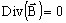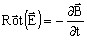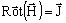• other equations: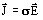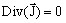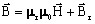## Fundamental dynamics equation in translating motion

The dynamics of a body in translating motion is expressed by the fundamental equation:

where:

• m is the mass of the moving body
• y is the instantaneous body position and ÿ is its linear acceleration
• Fr is the resistant mechanical force acting on the body
• Fem is the electromagnetic force acting on the body

## Fundamental dynamics equation in rotating motion

The dynamics equation for the rotating motion of a body is:

where:

• J is the moment of inertia of the rotating body
• θ is the body's angular position and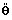is its angular acceleration
• Γr is the resistant mechanical torque acting on the body
• Γem is the electromagnetic torque acting on the body

## Solving principle

The magneto-mechanical coupling is a weak coupling between the electromagnetic and kinematic aspects of the problem. To solve this type of problem, we apply a four-stage procedure, as outlined below. At each time step, the electromagnetic aspect is analyzed first and then the kinematic one.

The algorithm of this method can be summarized as follows:

Stage Description
1 Solve the Maxwell equations and compute the electromagnetic force or torque acting on the moving part for a given relative position between the moving and fixed parts of the device
2 Solve the equation of moving part dynamics, compute the acceleration and speed of the moving part during a time step and compute the new position of the moving part for the next time step.
3 Move the moving part to the new position and (if necessary) re-mesh the displacement area.